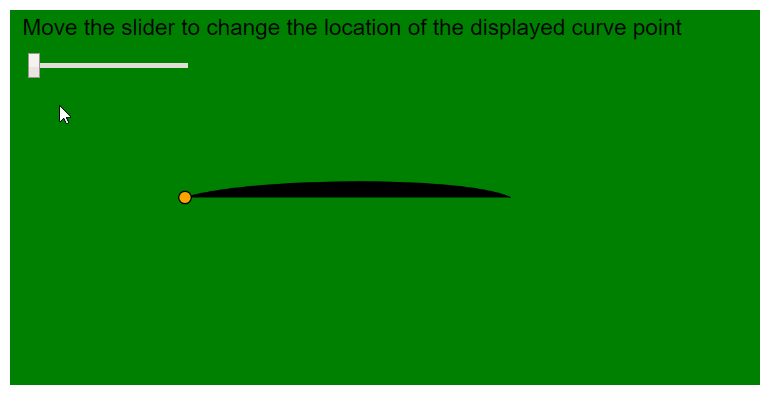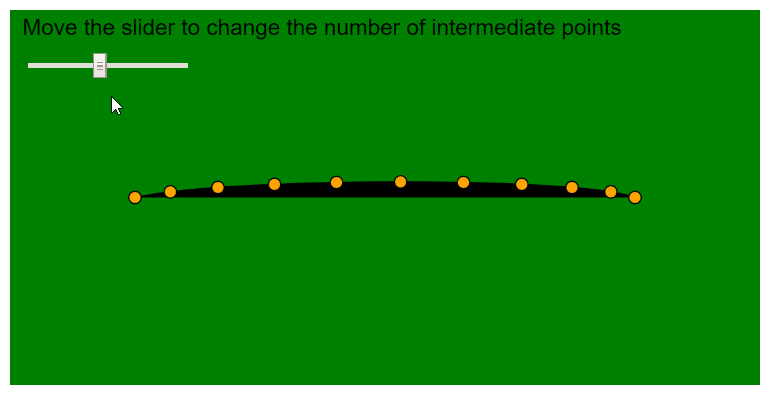# p5.js | curvePoint() Function

The curvePoint() function in p5.js is used to evaluate the coordinates of a curve at the given point. It takes in the coordinates of the curve for a particular axis and finds the coordinate of the curve at point “t”, which can be specified as a parameter.

The complete position of a point in the curve can be found out by finding using the function once on the x-coordinates and once on the y-coordinates of the curve and then using them together.

Syntax:

`curvePoint( a, b, c, d, t )`

Parameters: This function accepts five parameters as mentioned above and described below:

• a: It is a number that specifies the first point of the curve.
• b: It is a number that specifies the first control point of the curve.
• c: It is a number that specifies the second control point of the curve.
• d: It is a number that specifies the second point of the curve.
• t: It is a number between 0 and 1, which is used as the position between the beginning and ending of the curve coordinates.

Return Value: It returns a number which specifies the curve value at the given position.

The examples below illustrates the curvePoint() function in p5.js:

Example 1:

 `function` `setup() { ` `  ``createCanvas(600, 300); ` `  ``textSize(18); ` ` `  `  ``curvePointLocationSlider = createSlider(0, 1, 0, 0.1); ` `  ``curvePointLocationSlider.position(20, 40); ` `} ` ` `  `function` `draw() { ` `  ``background(``"green"``); ` `  ``fill(``"black"``); ` `  ``text( ` `    ``"Move the slider to change the location of the displayed curve point"``, ` `    ``10, 20 ` `  ``); ` ` `  `  ``// Get the required location of curve ` `  ``curvePointLocationValue = curvePointLocationSlider.value(); ` ` `  `  ``let p1 = { x: 50, y: 250 }; ` `  ``let p2 = { x: 140, y: 150 }; ` `  ``let p3 = { x: 400, y: 150 }; ` `  ``let p4 = { x: 350, y: 250 }; ` ` `  `  ``// Draw curve using curveVertex() ` `  ``beginShape(); ` `  ``curveVertex(p1.x, p1.y); ` `  ``curveVertex(p2.x, p2.y); ` `  ``curveVertex(p3.x, p3.y); ` `  ``curveVertex(p4.x, p4.y); ` `  ``endShape(); ` ` `  `  ``// Find the X and Y coordinate using the curvePoint() function ` `  ``let pointX = curvePoint(p1.x, p2.x, p3.x, p4.x, curvePointLocationValue); ` `  ``let pointY = curvePoint(p1.y, p2.y, p3.y, p4.y, curvePointLocationValue); ` `  ``fill(``"orange"``); ` ` `  `  ``// Display a circle at that point ` `  ``circle(pointX, pointY, 10); ` `} `

Output:Example 2:

 `function` `setup() { ` `  ``createCanvas(600, 300); ` `  ``textSize(18); ` ` `  `  ``maxPointsSlider = createSlider(2, 20, 10, 1); ` `  ``maxPointsSlider.position(20, 40); ` `} ` ` `  `function` `draw() { ` `  ``background(``"green"``); ` `  ``fill(``"black"``); ` `  ``text(``"Move the slider to change the number of intermediate points"``, 10, 20); ` ` `  `  ``// Get the required location of curve ` `  ``maxPoints = maxPointsSlider.value(); ` ` `  `  ``let p1 = { x: 50, y: 250 }; ` `  ``let p2 = { x: 100, y: 150 }; ` `  ``let p3 = { x: 500, y: 150 }; ` `  ``let p4 = { x: 350, y: 250 }; ` ` `  `  ``// Draw curve using curveVertex() ` `  ``beginShape(); ` `  ``curveVertex(p1.x, p1.y); ` `  ``curveVertex(p2.x, p2.y); ` `  ``curveVertex(p3.x, p3.y); ` `  ``curveVertex(p4.x, p4.y); ` `  ``endShape(); ` ` `  `  ``for` `(let i = 0; i <= maxPoints; i++) { ` `    ``// Calculate step using the maximum number of points ` `    ``let step = i / maxPoints; ` ` `  `    ``// Find the X and Y coordinate using the curvePoint() function ` `    ``let pointX = curvePoint(p1.x, p2.x, p3.x, p4.x, step); ` `    ``let pointY = curvePoint(p1.y, p2.y, p3.y, p4.y, step); ` `    ``fill(``"orange"``); ` ` `  `    ``// Display a circle at that point ` `    ``circle(pointX, pointY, 10); ` `  ``} ` `} `

Output:Online editor: https://editor.p5js.org/My Personal Notes arrow_drop_upCheck out this Author's contributed articles.

If you like GeeksforGeeks and would like to contribute, you can also write an article using contribute.geeksforgeeks.org or mail your article to contribute@geeksforgeeks.org. See your article appearing on the GeeksforGeeks main page and help other Geeks.

Please Improve this article if you find anything incorrect by clicking on the "Improve Article" button below.

Article Tags :

Be the First to upvote.

Please write to us at contribute@geeksforgeeks.org to report any issue with the above content.